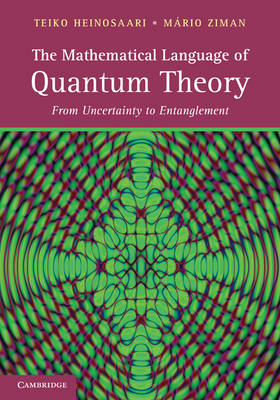home   cv   physics   teaching   photos Mathematical structures of quantum theory - Faculty of Mathematics, Physics and Informatics, Comenius University, Bratislava (5 credits) - code 2-FTF-227, winter semester (2+1h/week) - lecturer: Mário Ziman - undergraduate and graduate students - prerequisities: quantum mechanics, linear algebra, - room F2-125, Fridays 12:20-15:00 (subject to change) Content: § 1. Quantum Theory Refresher § 2. States and effects - basic statistical model of an experiment, density operators, convexity, pure and mixed states, purity § 3. Effects and superposition - duality, convexity, Gleason's theorem, double-slit interference § 4. Purification and automorphisms - tensor product, purification, group of automorphisms, Wigner's theorem § 5. Observables - POVM, convexity, sharp observables, § 6. Estimation and discrimination - informational completeness, minimum-error discrimination, unambiguous discrimination § 7. Quantum channels - complete positivity, Stinespring dilation theorem, § 8. Representations of quantum channels - Kraus decomposition, Choi-Jamiolkowski isomorphism, convexity § 9. Classes of channels - contractive, qubit channels, § 10. Time evolution - time, master equations, Schrodinger equation § 11. Instruments - quantum operation, projection "postulate", repeatability § 12. Measurement models - Stern-Gerlach apparatus, photodetector Exam: - homeworks (20 p + at least 5 bonus points), written test (10b) plus (optional) oral exam - A (>25 p), B(>20 p), C(>15 p), D(>10 p), E(>5 p) Literature: T.Heinosaari, M.Ziman: The Mathematical Language of Quantum theory (Cambridge, 2012) T.Heinosaari, M.Ziman: Guide to mathematical concepts of quantum theory, Acta Physica Slovaca 58, 487-674 (2008) Homeworks: Set 5 (ideally due 07/11/2014) 5.1 Consider a three-valued polarization observable F0=k |V⟩⟨V|, F+=k |+⟩⟨+|, F-=k |-⟩⟨-|, where |V⟩, |±⟩ = ± cosη|V⟩+ sinη|H⟩ are unit vectors (|V/H⟩ stands for vertical/horizontal polarization) and k is some positive constant. Find values of k and η for which these operators determines a valid POVM. (1p) 5.2 Illustrate the statistical map for the observable from the Task 5.1 above. (1p) Set 4 (ideally due 24/10/2014) 4.1 Consider a measurement apparatus being internally a uniform mixture of ideal Stern-Gerlach apparatuses oriented along the axis Z, or X. Find POVM description for such measurement. (1p) 4.2 Find conditions (in terms of its eigenvalues) under which an effect E can describe two different outcomes of the same measurement. (1p) Set 3 (ideally due 17/10/2014) 3.1 Is it possible to increase the purity of states by mixing them together? (1p) 3.2 Suppose you are give two sources. First producing systems in a state |ψ⟩ and the second producing systems in a state |φ⟩. How to check whether one of them is a superposition of the other. (1p) Set 2 (ideally due 10/10/2014) 2.1 Consider a physical system associated with two-dimensional Hilbert space. Find explicit range of parameters (in the basis of Pauli operators) determining the set of states. (1p) 2.2 Consider a physical system associated with two-dimensional Hilbert space. Find explicit range of parameters (in the basis of Pauli operators) determining the set of states. (1p) Set 1 (ideally due 03/10/2014) 1.1 Suppose we obtain a box containing 10 photons. We know that either there are 5 horizontally and 5 vertically polarized photons, or 5 left-circular and 5 right-circular polarized photons. Is it possible to say which box we received? If you have an answer, please describe why? (1p) 1.2 Let S be the swap operator acting on tensor product of isomorphic Hilbert spaces as follows S(|φ⊗ψ⟩)=(|ψ⊗φ⟩) on any pair of vectors |φ⊗ψ⟩. Show that for all operators ρ and ξ the following identity holds: trS(ρ⊗ξ)=tr[ρξ]. (1p) Bonus 1 What is measured by a realistic photon-resolving photodetector? In other words: determine effects describing the registration of N photons providing that the efficiency of detecting individual photons is η. (2p) Bonus 2 Use an observable from Taks 5.1. to discriminate between three pure states |V⟩, |+⟩, |-⟩. In particular, when an outcome Fj is observed then we conclude the state |j⟩ is prepared. Evaluate the probability of error? (2p) Bonus 3 Prove that measurements of position and momentum (using in a random manner) are not informationally complete. (2p)